# Triple Integral in Rectangular Coordinates Converting to Spherical Coordinates

## Homework Statement

Given that: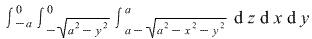Write an equivalent integral in spherical coordinates.

## Homework Equations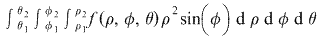(Triple integral in spherical coordinates.)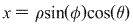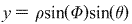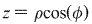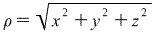(Conversions from rectangular to spherical coordinates.)

(What spherical coordinates entail)

## The Attempt at a Solution

The region is going to be the region of the lower part of a sphere with radius a moved up a units on the z-axis that lies in the -x and -y plane. The limits of integration for theta should be 0 to pi/2. Need assistance with limits for phi and rho.

Last edited by a moderator:

HallsofIvy
For each y, x ranges from $-\sqrt{a^2- y^2}$ to 0. $x= -\sqrt{a^2- y^2}$ gives $x^2+ y^2= a^2$, the circle with center (0,0) and radius a. Since x is the negative square root and we already know that we are in the lower half plane (y< 0), we are looking at the portion of that circle in the third quadrant.
For each (x,y), z ranges from $a- \sqrt{a^2- x^2- y^2}$ to a. $z= a- \sqrt{a^2- x^2- y^2}$ gives $x^2+ y^2+ (z-a)^2= a^2$, the sphere with center at (0,0,a) and radius a. Since z goes up to a, we are in the lower half sphere. That is, we are looking at the part of the sphere $x^2+ y^2+ (z-a)^2= a^2$ where x and y are negative and z< a.
I would be inclined to introduce new coordinates: x'= x, y'= y, z'= z- a. In those coordinates, the region we are integrating over is the "seventh octant" in which x, y, and z are all negative. To get that sphere, let $\rho$ go from 0 to a, $\theta$ from $\pi$ to $3\pi/2$, and $\phi$ from $\pi/2$ to $\pi$. The integrand is still "1" and the "differential of volume" is still $\rho sin^2(\phi)d\phi d\theta d\rho$. In fact, because the integrand is "1", this integral is just the volume of the region: 1/8 of the volume of a sphere of radius a and so is $(1/8)(4/3)\pi a^3= (1/6)\pi a^3$. You can use that as a check.